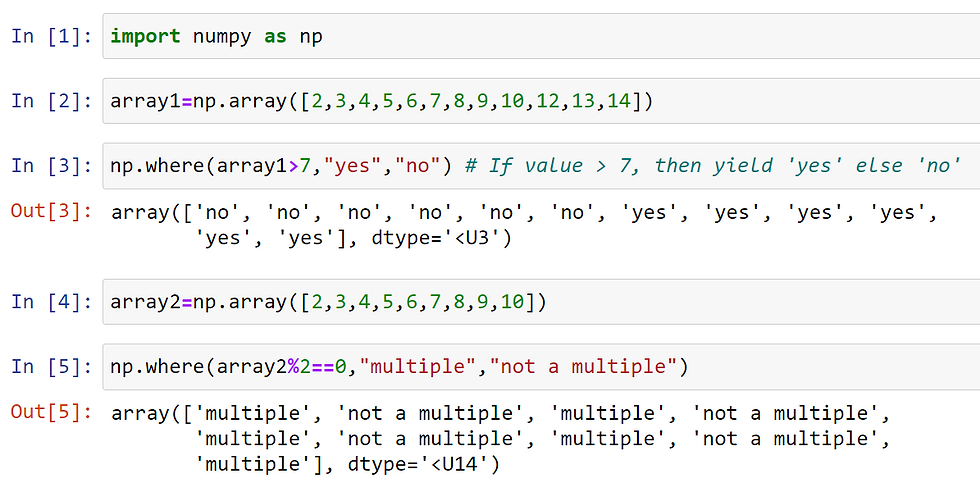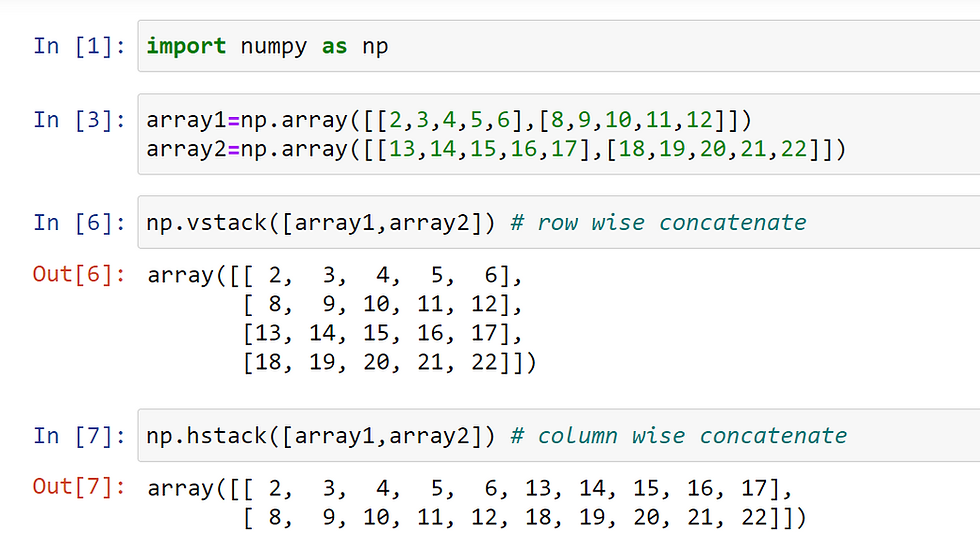top of page
Search

# NumPy Part 4

## Get The Index Locations That Satisfy A Particular Condition

• We use where() function to get the index location that satisfy a particular condition

• When we apply boolean indexing we can extract the elements from the array satisfying the condition

• But to get the location of these arrays satisfying a condition we use where() function

Example 1• Once you have the positions, you can extract them using the array’s take() method

Example 2• In np.where() we can also pass two more arguments(optional) they are x, and y

• This arguments works like an if-else statement. if condition is true then x is yielded or else y is yielded

Example 3• Sometimes we want to save this NumPy objects and load it back

• For saving the NumPy objects we use np.save() function

• When we want to store a single array store it as .npy file in np.save() function

• When we want to store multiple array in a single file store it as .npz file in np.savez() function

• np.save(filename to be stored,array)

• np.savez(filename to be stored,array)

• Use file attribute to load .npz files

Example 4## Concatenating two ndarrays

• NumPy provides two different ways to do concatenation

1. np.concatenate()

2. np.vstack() or np.hstack()

### np.concatenate([ndarray1,ndarry2],axis)

• By using np.concatenate() we can concatenate or combine two ndarrays

• By using np.concatenate() we can concatenate or combine two ndarrays column wise or row wise by changing the axis parameter to 0 and 1

Example 5### np.vstack() or np.hstack()

• By using np.vstack() we can stack vertically (rows wise concatenate similar to np.concatenate(axis=0))

• By using np.hstack() we can stack horizontally (column wise concatenate similar to np.concatenate(axis=1))

Example 6## How To Apply a function column wise or row wise

• To apply a user defined or built in function we can use apply_along_axis() function provided by numpy module

• apply_along_axis(function,axis,array)

• function-- function to apply

• axis- apply row wise or column wise

• array - which array this function should be applied

Example 7Example 8## How to Add A New Axis To A NumPy Array

• We can add a new axis to ndarray by using newaxis() function

• Whenever we need to convert a 1d to 2d or 2d to 3d ... we can use newaxis to convert to a higher dimensional ndarray

• we can convert by adding a newaxis column wise or row wise

Example 9## How To Sort An Array

• We can use sort() to sort an array column wise or row wise

• To sort an array column wise or row wise use axis = 0 or 1

Example 10• np.argsort() can be used to return the index positions that would sort the array

Example 11